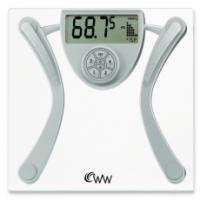# Kilograms to Pounds

People often find the need to convert one unit of weight to another. Therewill usually come moments where one has to convert kilograms to pounds. Conventional and everyday usage would have you use 2.2 pounds for every single kilogram. However this rounded off amount isn’t that accurate. A more accurate conversion from one kilogram is 2.20462262 pounds.

### Conventional Formula

Here is the conventional formula that ordinary folks use to convert kilograms to pounds:

### Amount in Kilograms x 2.2 = weight in pounds

Now, if you want to calculate the weight from pounds to kilograms you divide the number of pounds by 2.2 then you get the amount in kilograms. So for example a woman that weighs 70 kilograms will weigh 154 pounds given the said formula. And, turning things around, a man weighing 110 pounds will weigh 50 kilograms.

The formula is easy enough to follow. With some practice, you’ll get used to short exercise in mathematics converting from kilograms to pounds. However, if you tinker around and let a little more curiosity work then you will wonder where in the world did we get 2.2, or 2.20462262?

### Digging Definitions

If one digs a bit deeper, you’ll find that there are discrepancies in the calculations in theory. The discrepancies are due to the nature of both kilograms and pounds since they measure two different things. One of these two is actually a measure of weight while the other is a measure of mass. Ordinary folks would simply just think of both of them as measures of weight but clearly they are not the same.

The word pound comes from the Latin ‘pendere’, which basically translates to ‘to weigh’. This unit of measure is today’s staple for many weight systems used in many parts of the world. You might have also wondered why people abbreviate pounds to ‘lb’. That also has roots in Latin, from the word ‘libra’ to be exact. Libra can be translated into either ‘balances’ or ‘scales’. Historically, it is used to describe the unit of pound force used in the Roman Empire. Thus, today we abbreviate pounds into ‘lb’, signifying libra.

On the other hand, kilogram is a measure of mass and is part of today’s standard metric system. The kilogram is defined to be the exact mass of one liter of water with a temperature of four degrees Celsius. However, in the household, people just define a kilogram as a measure of weight not knowing that it is actually a measure of mass. For this reason, there is no exact conversion for kilograms to pounds since what you are doing is just translating one measurement to the other.

### Tapping the Inner Geek

Those who are not satisfied with pounds being a unit of force/weight and kilograms as a measure of mass will have to dig into how two differing units of measurement are converted, going from kilograms to pounds. The weight in newtons will be the equivalent of mass x g. A pound of force will be 4.448 newtons to be exact. You get the rounded off 2.2 pounds when you take 9.801 m/s^2 divided by 4.448 newtons/pound.